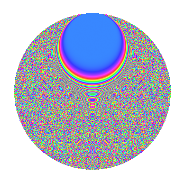# Properties

 Label 2016.2.gdLevel 2016 Weight 2 Character orbit gd Rep. character $$\chi_{2016}(155,\cdot)$$ Character field $$\Q(\zeta_{24})$$ Dimension 2304 Sturm bound 768

# Related objects

## Defining parameters

 Level: $$N$$ = $$2016 = 2^{5} \cdot 3^{2} \cdot 7$$ Weight: $$k$$ = $$2$$ Character orbit: $$[\chi]$$ = 2016.gd (of order $$24$$ and degree $$8$$) Character conductor: $$\operatorname{cond}(\chi)$$ = $$288$$ Character field: $$\Q(\zeta_{24})$$ Sturm bound: $$768$$

## Dimensions

The following table gives the dimensions of various subspaces of $$M_{2}(2016, [\chi])$$.

Total New Old
Modular forms 3104 2304 800
Cusp forms 3040 2304 736
Eisenstein series 64 0 64

## Trace form

 $$2304q + O(q^{10})$$ $$2304q - 48q^{27} + 40q^{30} + 64q^{36} - 48q^{39} + 104q^{48} + 312q^{50} - 72q^{58} + 56q^{60} + 128q^{66} - 120q^{72} + 48q^{76} - 24q^{78} + 112q^{87} - 120q^{90} - 456q^{92} - 64q^{96} + 128q^{99} + O(q^{100})$$

## Decomposition of $$S_{2}^{\mathrm{new}}(2016, [\chi])$$ into newform subspaces

The newforms in this space have not yet been added to the LMFDB.

## Decomposition of $$S_{2}^{\mathrm{old}}(2016, [\chi])$$ into lower level spaces

$$S_{2}^{\mathrm{old}}(2016, [\chi]) \cong$$ $$S_{2}^{\mathrm{new}}(288, [\chi])$$$$^{\oplus 2}$$

## Hecke Characteristic Polynomials

There are no characteristic polynomials of Hecke operators in the database